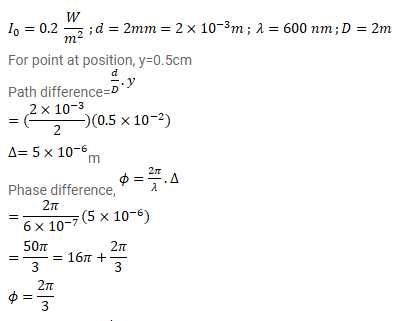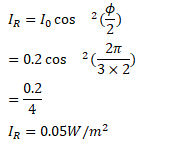# In a Young's double slit experiment, the separation betweenQuestion:

In a Young's double slit experiment, the separation between the slits $=2.0 \mathrm{~mm}$, the wavelength of the light=600 $\mathrm{nm}$ and the distance of the screen from the slits $=2.0 \mathrm{~m}$. If the intensity at the centre of the central maximum is $0.20 \mathrm{~W} / \mathrm{m}^{2}$, what will be the intensity at a point $0.5 \mathrm{~cm}$ away from this centre along the width of the fringes?

Solution: